Next: The NonLinear procedure Up: Solving systems with linear Previous: Mathematical background   Contents

The procedure is implemented as:

int Solve_Simplex(int m,int n,int NbNl,
INTEGER_VECTOR TypeEq,
INTERVAL_VECTOR (* IntervalFunction)(int,int,INTERVAL_VECTOR &),
void (* NonLinear)(INTERVAL_VECTOR &x,INTERVAL_VECTOR &X),
void (* CoeffLinear)(MATRIX &U),
double MaxDiam,
int FullSimplex,
INTERVAL_VECTOR & TheDomain,
int Order,int Iteration,int Stop,
double epsilon,double epsilonf,double Dist,
INTERVAL_MATRIX & Solution,int Nb,
int (* Simp_Proc)(INTERVAL_VECTOR &))
the arguments being:
• m: number of unknowns
• n: number of equations, see the note 2.3.4.1
• NbNl: number of equations that have no linear term at all or are inequalities. If you omit this parameter its value will be assumed to be 0 and you have to omit the TypeEq parameter.
• TypeEq: an array of integers that indicate the type for the equations. TypeEq(i) is -1,0,1 if equation i is an inequality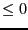, an equation or an inequality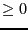.
• IntervalFunction: a function which return the interval vector evaluation of the equations, see the note 2.3.4.3 on how to write this procedure. The equations must be ordered: first the equations with linear terms then the equations without any linear terms and finally the inequalities
• NonLinear: a procedure to compute the non linear part of the equations, see note 2.14.2.1
• CoeffLinear: a procedure that return a matrix which contain the constant coefficients of the linear term in the equation, see note 2.14.2.2
• MaxDiam: the simplex method will not be used on boxes whose maximal width is lower than this value. Should be set to 0 or a small value
• FullSimplex: this flag is used to indicate how much we will use the simplex method (which may be costly). If set to -1 only the phase I of the simplex will be used. If set to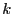with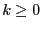, then the full simplex method will be used recursively on the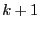variables having the largest interval width.
• TheDomain: box in which we are looking for solution of the equations
• Order: the type of order which is used to store the intervals created during the bisection process. This order may be either MAX_FUNCTION_ORDER or MAX_MIDDLE_FUNCTION_ORDER. See the note on the order 2.3.4.4.
• M: the maximum number of boxes which may be stored. See the note 2.3.4.5
• Stop: the possible values are 0,1,2
• 0: the algorithm will look for every solution in TheDomain
• 1: the algorithm will stop as soon as 1 solution has been found
• 2: the algorithm will stop as soon as Nb solutions have been found
• epsilon: the maximal width of the solution intervals, see the note 2.3.4.6
• epsilonf: the maximal width of the equation intervals for a solution, see the note 2.3.4.6
• Dist: minimal distance between the middle point of two interval solutions, see the note 2.3.4.7
• Solution: an interval matrix of size (Nb,m) which will contained the solution intervals. This list is sorted using the order specified by Order
• Nb: the maximal number of solution which will be returned by the algorithm
• Simp_Proc: a user-supplied procedure that take as input the current box and proceed to some further reduction of the width of the box or even determine that there is no solution for this box, in which case it should return -1 (see note 2.3.3). Remember that you may use the 3B method to improve the efficiency of this algorithm (see section 2.3.2).

Note that the Simp_Proc argument may be omitted.

SubsectionsNext: The NonLinear procedure Up: Solving systems with linear Previous: Mathematical background   Contents
Jean-Pierre Merlet 2012-12-20